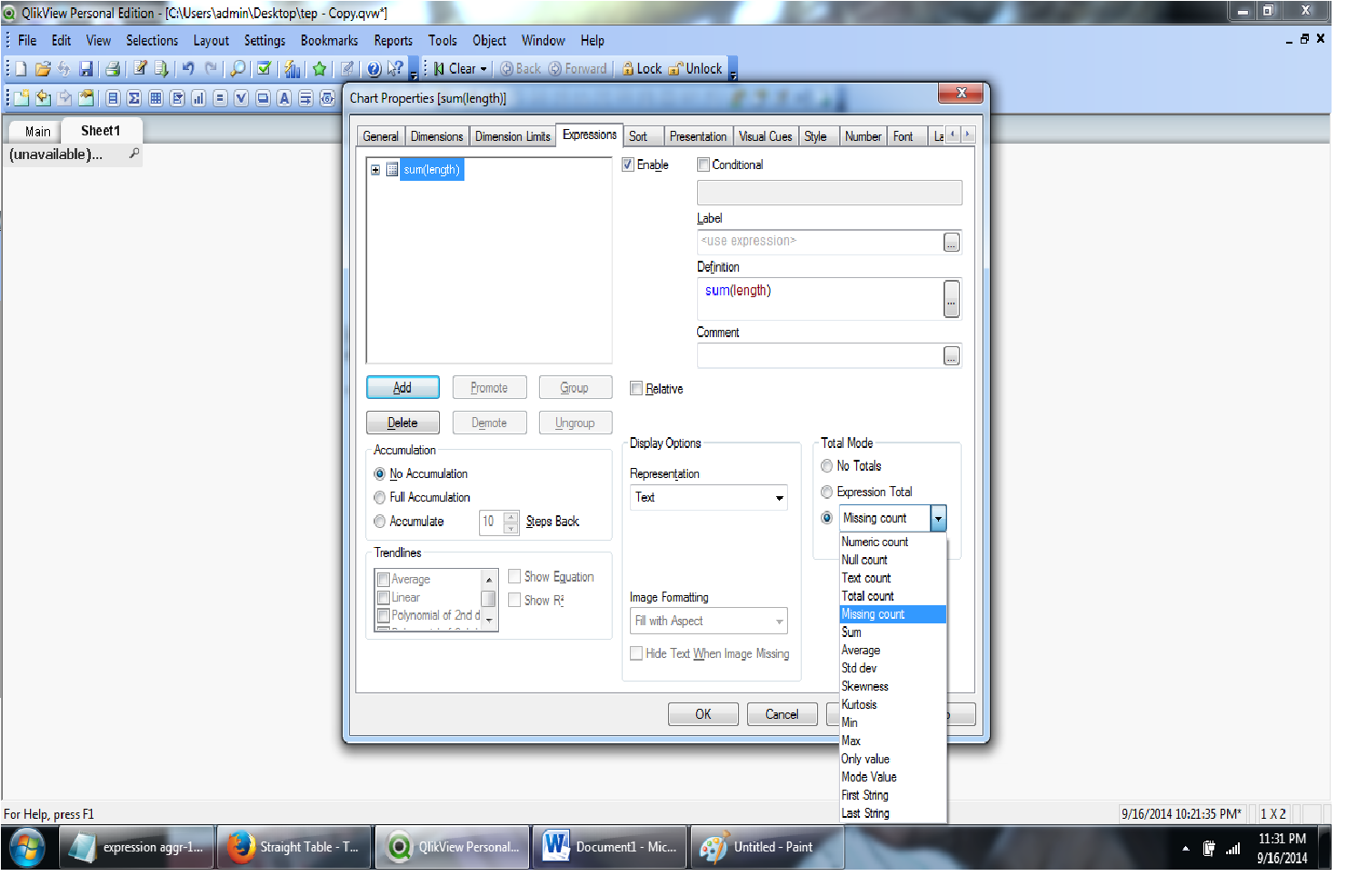# New to QlikView

Discussion board where members can get started with QlikView.Not applicable

## Straight Table - Total need custom total

Hello,

I am new in the Qlikview tool, I have straight table where grid rows calculation has sum calculated measures but in the "Totals" don't want the sum of the measures in my grid rows. But need apply the new calculation. Is it possible  and how can we do it. Since don't find any custom totals in the table properties.

Tags (4)
8 Replies
Honored Contributor II

## Re: Straight Table - Total need custom total

Can you upload file QV ?

And explain more details that you need, please.

TX

New Contributor III

## Re: Straight Table - Total need custom total

What is "the new calculation" you're trying to apply for the 'Total'?

Would a pivot table work better for you or do you need a straight table for your end goal?

Valued Contributor II

## Re: Straight Table - Total need custom total

We have some predefined options... It cannot be customized..Hope this helps.

-Sundar

Valued Contributor II

## Re: Straight Table - Total need custom total

You can use a statement like:

if(dimensionality()=0, avg(Value), sum(Value))

where avg(Value) would be the expression for your total.

Hope this helps!Not applicable

## Re: Straight Table - Total need custom total

I am uploading the QVW. SO I have two List box in the sheet. If user select first one BU LVL1 I have different calculation, like remove the one of the column in sum I am doing but in straight table has BU LVL 2 description so that it using different calculation but the total is actually for all BU LVL2 . So it has to calculate at BU LVL 1 so need different calculation.Not applicable

## Re: Straight Table - Total need custom totalNot applicable

## Re: Straight Table - Total need custom total

Hi Viral,

Why dont you keep both the dimensions in the chart and enable/disable them based on selections?

So if BU LVL1 is selected, the chart will have BU LVL1 as the active dimension and also Total up based on that dimension.

Would that help or am I missing something?

SK

Highlighted
New Contributor